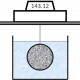# Micro-annulus – importance for hydraulic isolation###### What is expected from the cement in cementing for the life of the well?
April 1, 2021###### The membrane test – experimental considerations
June 7, 2021

In a previous article I discussed the expected volumes, pressures, and injection rates during repairs of a micro-annulus ranging from 10 to 100 μm in thickness.  In this article I demonstrate the importance of a micro-annulus compared with the permeability of the cement sheath for zonal isolation.

Consider the situation above with a micro-annulus (indicated in red) with a width w around a casing of diameter 2RC cemented in a hole of diameter 2RH.  With a differential pressure ΔP applied across a length L of the micro-annulus the flow rate Q, of an incompressible Newtonian fluid of viscosity μ, through a micro-annulus is given by :

Now, assume that there is no micro-annulus and the flow rate Q is through the matrix of the cement sheath under the same conditions (ΔP, L, μ) but the flow area (A) is that through the cement between the casing and formation as shown below.

The permeability of the cement sheath, k, is given by:

Substituting in equation 3 for Q (Equation 1) and A (Equation 2), the equivalent permeability of the cement sheath to give the same flow rate as a micro-annulus of width w is given by:

## Units

The equations above are for SI units: length (m), area (m2), flow rate (m2/s), pressure (Pa), viscosity (Pa.s) and permeability (m2).

## Results

The easiest way to use Equation 4 is to convert the micro-annulus width (conventionally given in microns) and casing and hole diameters (conventionally given in inches) to metres.

NOTE: that in Equation 4 the casing and hole sizes are the radii not diameters.

The calculated permeability (in m2) can then be converted back to the unit Darcy.

Results for two casing/hole sizes and for micro-annuli up to 100 microns are shown in the figure below.  The horizontal red line indicates the permeability of 0.1 mDarcy, which is generally considered the highest permeability of a material for zonal isolation.

## Conclusion

The presence of micro-annuli with widths above about 3 microns leads to an effective permeability of the casing-cement-rock (or casing) system >0.1 mDarcy.  Note that the surface roughness of casing is typically at least 10 microns and the resulting tortuous paths from the smaller micro-annuli have not been considered.  The effective permeability is slightly affected by the cross-sectional area of the annular section.

The formation of micro-annuli should be prevented to minimise the risk of loss of zonal isolation.